# MCQs on Power Systems

##### Page 32 of 67. Go to page 1 2 3 4 5 6 7 8 9 10 11 12 13 14 15 16 17 18 19 20 21 22 23 24 25 26 27 28 29 30 31 32 33 34 35 36 37 38 39 40 41 42 43 44 45 46 47 48 49 50 51 52 53 54 55 56 57 58 59 60 61 62 63 64 65 66 67
01․ In which of the following given faults, all the sequence currents are equal?
LG
LLG
LLL
LL

Assumptions in LG faults are 1. Fault current If = IR 2. IY = IB = 0 3. Vf = IR×Zf = If×Zf Therefore,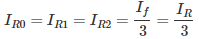Where, IR0, IR1 and IR2 are sequence currents.

02․ If all the sequence voltages at the fault point in a power system are equal, then the fault point is a
LLL
LL
LG
LLG

In double line to ground fault, all the sequence networks are connected in parallel. Therefore, all the sequence voltages at the fault point are equal. VR0 = VR1 = VR2 Where, VR0, VR1 and VR2 are sequence voltages.

03․ The zero sequence current of a generator for line to ground fault is j3 pu. Then the current through the neutral during the fault is
j3 pu
j1 pu
j9 pu
j6 pu

Assumptions in LG faults are 1. Fault current If = IR 2. IY = IB = 0 3. Vf = IR×Zf = If×Zf Therefore,Therefore, Current through the neutral during the fault If = 3×IR0 If = 3× j3 = j9 pu

04․ Which of the following statement is/are true?Zn
The neutral grounding impedance Zn appears as 3Zn in zero sequence equivalent circuit
The neutral grounding impedance Zn appears as Zn in zero sequence equivalent circuit
both 1 and 2
nether 1 nor 2

A fault current of 3 IR0 produces a drop of 3 IR0× Zn and to show in the equivalent zero sequence network the same drop where current of IR0 flows, the impedance should be 3 Zn. Therefore, the neutral grounding impedance Zn appears as 3 Zn in zero sequence equivalent circuit.

05․ A fault occurring at the terminals of an unloaded synchronous generator operating at its rated voltage has resulted in the following values of currents and voltages. IR0 = j 2.37 pu IR1 = -j 3.05 pu IR2 = j 0.68 pu VR0 = VR1 = VR2 = 0.237 pu. Which of the following faults has occurred?
LL
LG
LLG
LLL

In double line to ground fault, all the sequence networks are connected in parallel. Therefore, all the sequence voltages at the fault point are equal.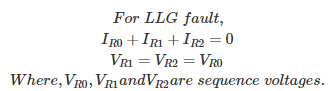06․ The line currents of 3-phase supply are: IR = 3 + j 5 A IY =2 + j 2 A IB = -2 - j 1 A The zero sequence current will be
1 + j 2 A
5 + j 7 A
1 + j 4 A
-2 - j 1 A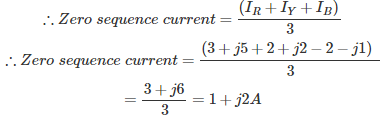07․ An unloaded generator with a pre-fault voltage 1 pu has the following sequence impedance: Z0 = j 0.15 pu, Z1 = Z2 = j 0.25 pu The neutral is grounded with a reactance of 0.05 pu. The fault current in pu for single line to ground fault is
7.25 pu
2.5 pu
3.75 pu
4.28 pu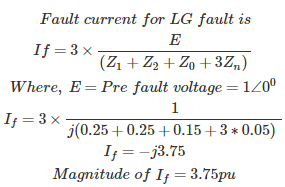08․ A power system network has n nodes, Z33 of its bus impedance matrix is j0.5 pu. The voltage at node 3 is 1.3∠ -10°. If capacitor is having reactance of -j3.5 pu is now added to the network between node 3 and reference node, the current drawn by the capacitor in pu is
0.325∠ -100°
0.725∠ 80°
0.371∠ -100°
0.433∠ 80°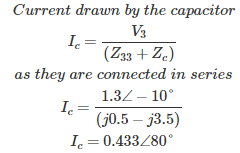09․ In a 3-phase, 5 kV, 5 MVA system, what is the base impedance?
5 ohms
500 ohms
0.5 ohm
50 ohms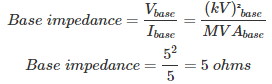10․ When is the ferranti effect on long overhead lines experienced?